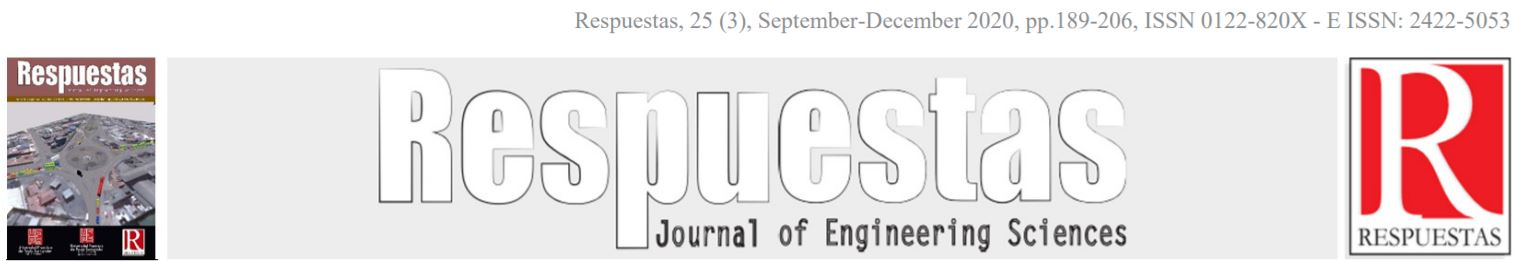Articulo Originalhttps://doi.org/10.22463/0122820X.2803

# Simulacion del Control Nivel y Presion en el Domo de una Caldera Acuotubular usando Control Station® (LOOP-PRO)

## Simulación del Control Nivel y Presión en el Domo de una Caldera Acuotubular usando Control Station® (LOOP- PRO)

#### Orlando J. Gasca-Taba11* Fiderman Machuca-Martínez2*

1*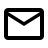Escuela de Ingeniería Química, gascataba@msn.com , Universidad del Valle, Cali, Colombia,

2*Escuela de Ingeniería Química, fiderman.machuca@correounivalle.edu.co , Universidad del Valle, Cali, Colombia,

How to cite: O.J. Gasca-Taba, F. Machuca-Martínez, “Simulacion del Control Nivel y Presion en el Domo de una Caldera Acuotubular usando Control Station® (LOOP-PRO)”. Respuestas, vol. 25, no. 3, pp. 189-206, 2020.

© Peer review is the responsibility of the Universidad Francisco de Paula Santander. This is an article under the license CC BY-NC 4.0.Approved: October 23, 2020.

Keywords

Control Station, Boilers, Simulation, controllers tuning, PID, IMC.

Abstract

The present work consisted of the simulation of the automatic control in the dome of a water tube boiler, to know in detail the behavior of the level and pressure and its influence on the operation of the boiler. To obtain the dynamics of each of the variables in the dome, the boiler software was used. Different disturbances were performed in manual mode to collect data and later fit them to a dynamic model. With the adjusted functions, a 2x2 MIMO multivariable control strategy was proposed, which was validated with the relative gain criterion proceeding to the decoupling methodology.
The parameters of each controller were tuned by the BLT method for multivariate systems and validated with the Nyquist stability criterion. The 2x2 system was simulated in the Control Station software with the controllers in automatic mode and step-type disturbances were performed to observe the influence that each parameter of the PID controller has on the response time, oscillation and offset of the controlled variables and their reflection on the other variables. With the criteria of overshoot and rise time, each parameter was retuned.
To ensure that the dynamics in the dome remains controlled, disturbances were made for servo control and regulator control, obtaining satisfactory results for each system. PID controllers were shown to respond quickly and efficiently.

Palabras claves

remoción de color, fibras de fique, nanopartículas de óxido de zinc, síntesis in situ, impregnación ex situ, carmín índigo.

Resumen

El presente trabajo consistió en la simulación del control automático en el domo de una caldera acuotubular, para conocer en detalle el comportamiento del nivel y presión y su influencia en la operación de la caldera. Para obtener la dinámica de cada una de las variables en el domo, se utilizó el software de calderas. Se realizaron diferentes perturbaciones en modo manual para recolectar datos y posteriormente ajustarlos a un modelo dinámico. Con las funciones ajustadas, se propuso una estrategia de control multivariable MIMO 2x2, que se validó con el criterio de ganancia relativa procediendo a la metodología de desacople.
Los parámetros de cada controlador fueron sintonizados por el método BLT para sistemas multivariables y validados con el criterio de estabilidad de Nyquist. Se simuló el sistema 2x2 en el software Control Station con los controladores en automático y se realizaron perturbaciones tipo escalón para observar la influencia que tiene cada parámetro del controlador PID en el tiempo de respuesta, oscilación y offset de las variables controladas y su reflejo en las demás variables. Con el criterio de overshoot y rise time se volvió a sintonizar cada parámetro.
Para garantizar que la dinámica en el domo se mantenga controlada, se realizó perturbaciones para servo control y control regulador obteniendo resultados satisfactorios para cada sistema. Se demostró que los controladores PID responderán de manera rápida y eficiente.

Introduction

Since the invention of the steam engine in 1769 by Dionysius Papin and further developed by James Watt in 1776, the steam engine has been the study of many. The first boilers were simply airtight metal vessels containing water in which a flame was lit underneath to heat them to produce steam. As welding and riveting techniques advanced, so did the boilers, but a major problem remained: they exploded due to the high pressure inside the metal container or the improper mixing of fuel to generate flame.

To remedy this problem it was necessary to develop control systems in the boilers, starting with a simple manual control which included a barometer observed by a person who knew that if it passed a certain pressure, had to decrease the flame. Up to the new automatic and digital control systems that control pressure, temperature, water flow and fuel mixture in continuous boilers .

In industry it is common to find processes that consist of two or more input and output variables. Manipulating one variable can affect one or more controlled variables or vice versa, requiring a more complex control system ,,,,.

A typical example is the case of a water-tube boiler that, by varying the fuel mixture or the water feed flow to the boiler, generates disturbances in the dome (height), increasing or decreasing steam production depending on the disturbance and being reflected in the internal pressure of the dome, which increases the risk of explosion and requires MIMO control systems. The control of these two manipulated variables (combustion mixture and water supply to the boiler) allows the boiler to be operated efficiently and safely, minimizing the risk of accidents. Therefore, simulation and process control through the use of software is one of the areas of greatest development due to its implications in the education and training of personnel ,,,.

The control of equipment associated with the operation of boilers is of vital importance as it is one of the fundamental industrial services for the performance of the chemical industry , , . Due to its importance and complexity there are several ways to control the various variables of a boiler: Thus, Koppel et al use a method of decoupling controllers using the method of relative gains for interacting systems , He and Tan use an adaptive algorithm to calculate the parameters of a PID controller and make a comparison of the performance of the controllers , Pan et al use a genetic algorithm to optimize the performance of the controllers to variable loads in the control system . On the other hand, Chen et al show a control strategy based on a third-order identification model for the optimization of a PID using the particle optimization methodology .

The use of Nyquist and Bode diagrams is fundamental for the understanding.

The present work deals with the solution of a 2x2 system for the modeling and simulation of a control system in the dome of a boiler using the Control Station software (LOOP PRO), where the technique of decoupling controllers is used to study the dynamics of the controllers and variables to be selected.

Materials and methods

Data collection from the boiler software.

To find the dynamics of each of the variables in the boiler dome, the boiler software  was used to perform perturbations of different types (step, ramp, double pulse, etc.) in manual mode and collect the data for each one (Figure 1). The Desing Tool of the Control Station software was used to fit the data to a dynamic model of first order plus dead time or higher order plus dead time depending on its approximation.

Variable matching.

With the functions fitted to a dynamic model, a 2x2 MIMO multivariable control strategy was proposed. Using the relative gain criterion, it was validated if the pairing of the variables is adequate and depending on the classification of the result (λ>1, λ<1, λ=1) we proceeded to follow with the decoupling methodology . The variables proposed for the study are (Figures 1 and 2): manipulated, Inlet water mass flow (QF) and Fuel flow rate (QC); controlled, Dome water level (NW) and Steam outlet pressure (P). To complement the boiler dynamics, a disturbance variable was chosen: Steam outflow (QS).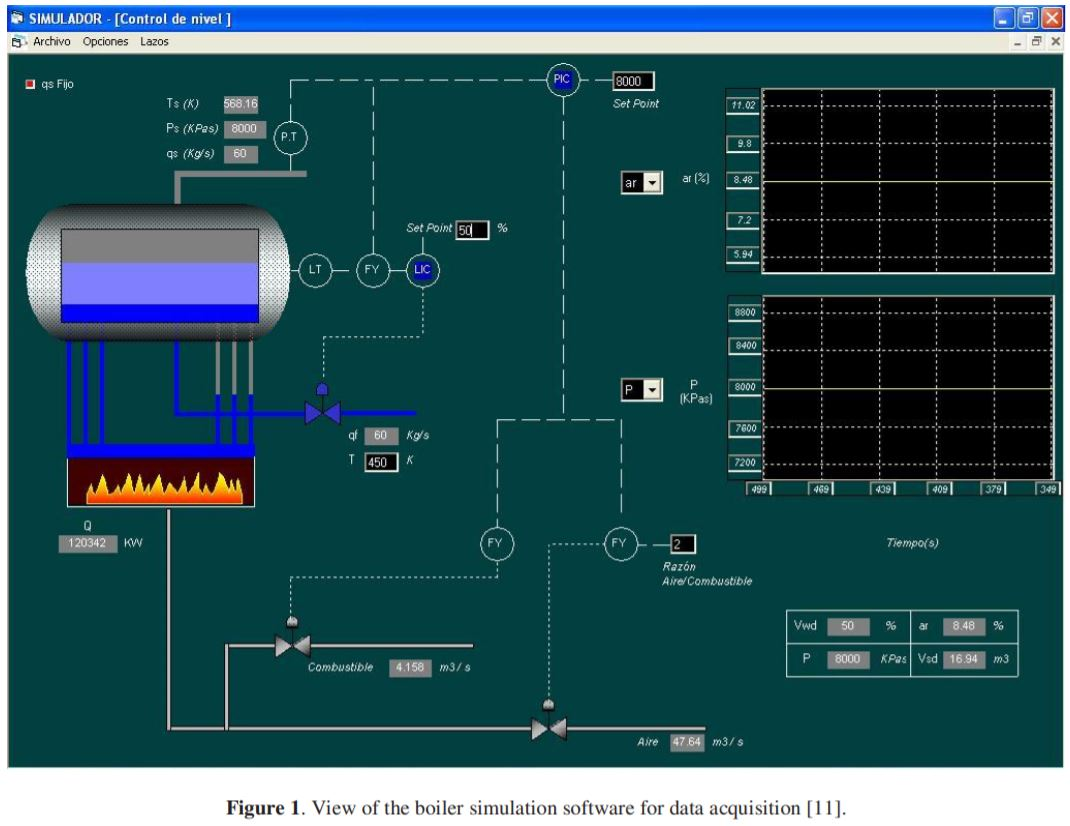Tuning of the controllers.

The controllers were tuned by the BLT method for multivariable systems, obtaining the initial parameters of each controller by the IMC method  for the main loops and thus, varying each parameter of the PI or PID controller until obtaining a Nichols plot, for each loop, that tangentially passes through the +2 dB curve. The parameters obtained by the BLT method were validated with the Nyquist stability criterion.

After obtaining and validating the parameters of each controller by the BLT method, the 2x2 system was simulated in the Control Station software (Loop Pro) to obtain the performance of each controller in its loop and interaction. To optimize each controller, each controller parameter (KC, τI and τD) was modified (increasing and decreasing) and the response velocities in each loop to a step perturbation at the setpoint were observed. With the step figure, the values of rise time and overshoot, each parameter of the PID controller was retuned obtaining short response times, with little oscillation and minimizing the offset in each loop.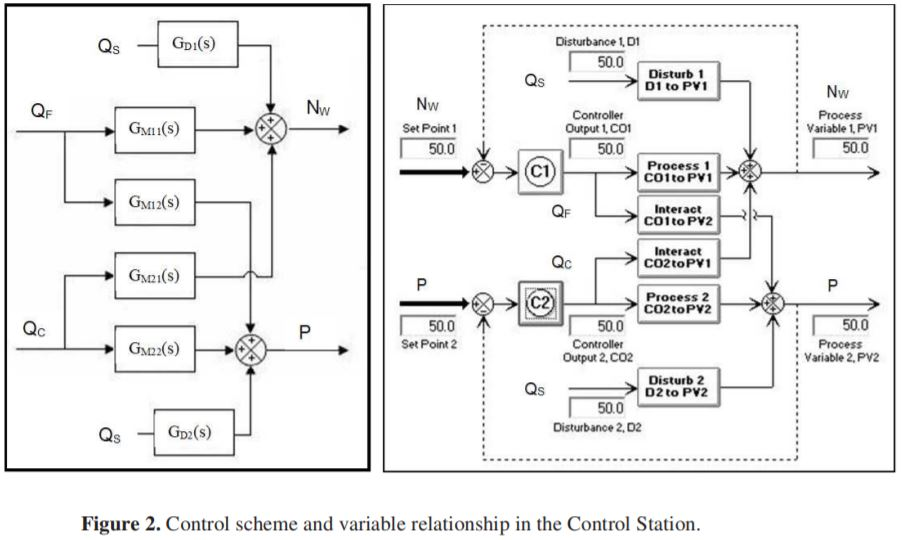Testing for servo and drive systems.

With the parameters obtained in the tuning, double rectangular pulse type tests were performed for the servo and controller system. Ensuring that the dynamics in the boiler dome will not be affected by any change in any of its variables and that the controllers will respond quickly and efficiently keeping each variable at its desired value  .

Control strategies and model characterization

The Control Station software interface (Figure 2) shows the strategy to simulate the automatic control of the process variables (NW - m3, P - Kpa) of a 2x2 multivariable system, from step, ramp, oscillatory or double rectangular pulse type changes in the setpoint of the manipulated variables (QF - Kg/s, QC - m3/s). Two interacting PID controllers are available to carry out the control of the 4 feedback loops described in the process, plus the perturbation (QS - Kg/s). In the case of the variable Nw, dome level, the volume of the system in m3 is specified for this case, which is given in % level.

The Control Station software (LOOP_PRO) has a design tool for obtaining the transfer function of the process, from the data collection of the variables affected by the disturbance. It is a tool with a feasible approach that simplifies the calculations of complex processes such as those of a boiler. The software uses the method of adjustment by summation of the squared error and its adjustment index R2. In order to reduce the error by the approximation of the software method, a suitable type of perturbation was chosen for any order. Obtaining greater pressure with higher orders.

One of the most practical and interesting applications of frequency response is the use of the pulse test to determine the transfer function of the process, instruments and other control devices . The double rectangular pulse test is one of the most used in the industry for its flexibility in the execution time, flexibility that does not have the sinusoidal test, their execution times can be long or short depending on the influence of the manipulated variable on the controlled. It is accurate in higher order models and generates a complete Bode diagram. The only requirement of this test is to reach the initial steady state point .

Obtaining the dynamics in the dome.

The type of disturbance selected is the double rectangular pulse. In order to obtain the complete dynamics of the boiler dome, this test was performed for each manipulated variable to see its effect on each process variable and thus obtain its transfer function. This procedure was performed for direct processes, interactions and perturbations. As an example, the case of inflow (QF - Kg/s) - water volume (NW - m3) is taken (Figure 3). The transfer functions obtained by the Control Station software (Table 1) resemble the behavior of the boiler simulator; for this reason, they comply with the dynamics of a boiler dome with an approximation of R2 ranging from 0.9 to 0.99.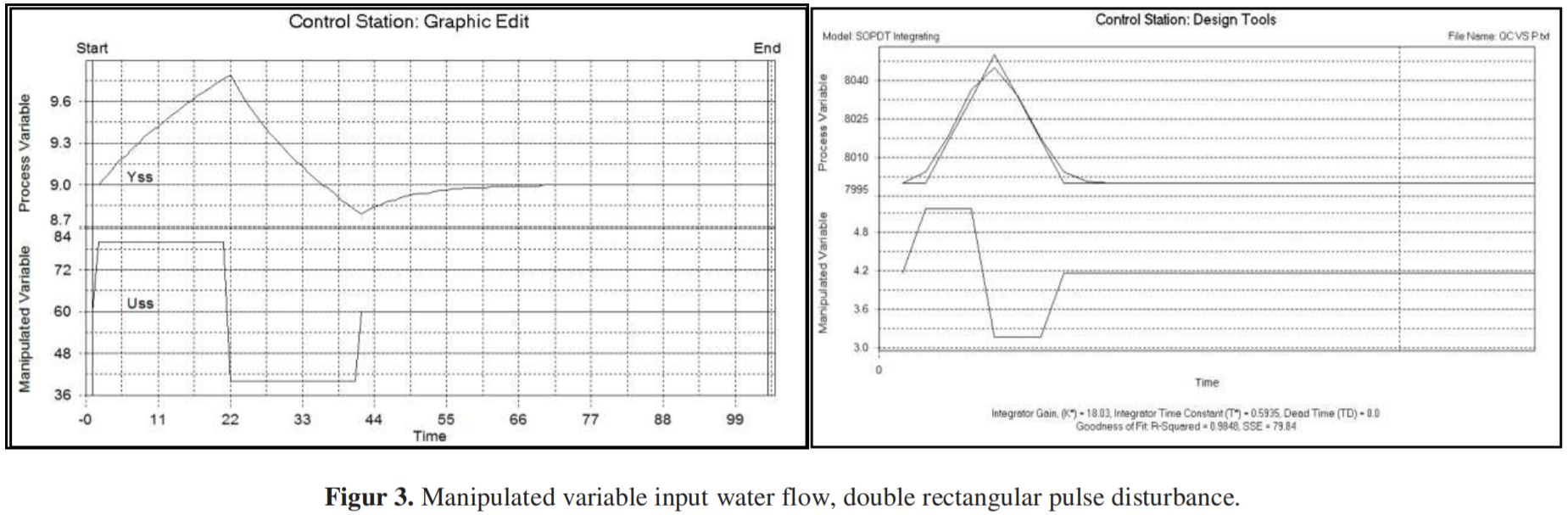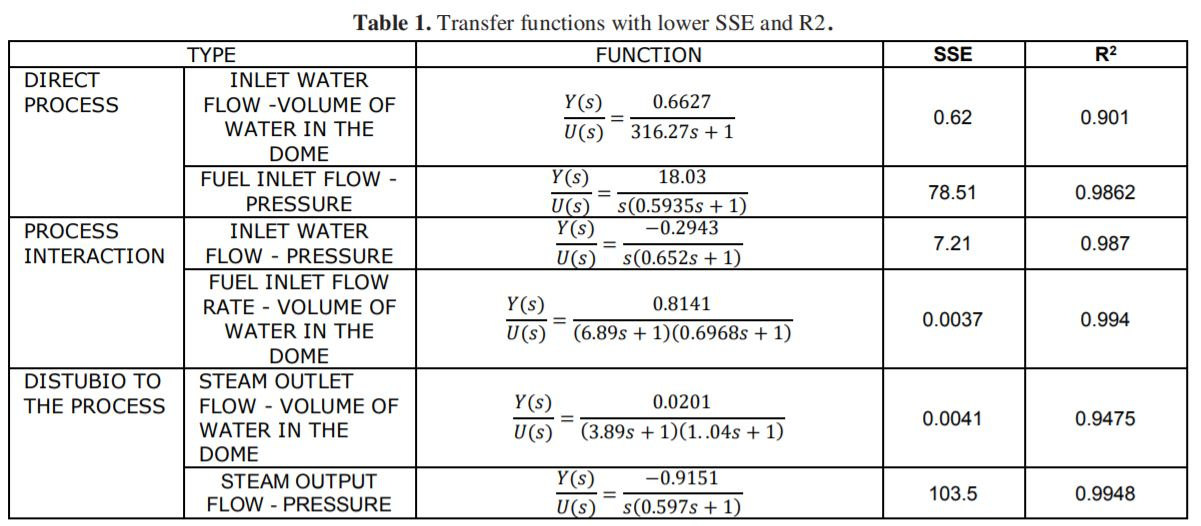Each of the responses or changes shown in the controlled variables caused by the perturbations made to the manipulated or disturbance variables, show a typical behavior of the real process inside the dome of a boiler and its combustion, i.e., by increasing the feed flow, the liquid level in the dome increases which in turn decreases the pressure, and by decreasing its level also decreases and increases the pressure. By increasing the fuel flow, the pressure increases which in turn increases the level due to the increase in the size of the vapor bubbles (Expansion) and by decreasing it, the pressure decreases which in turn decreases the level due to the contraction phenomenon. By increasing the vapor outflow, there is a significant pressure drop and an increase in the liquid level due to its loss of pressure and mass.

Matching manipulated variables with controlled variables for each control loop

To begin with, it makes sense that each controlled variable is controlled by the manipulated variable that exerts the most "influence" on it. In this sense, influence and gain have the same meaning and, consequently, in order to make a decision, all the gains of the steady state process (4 gains for a 2x2 MIMO system) must be found. Specifically, these gains are: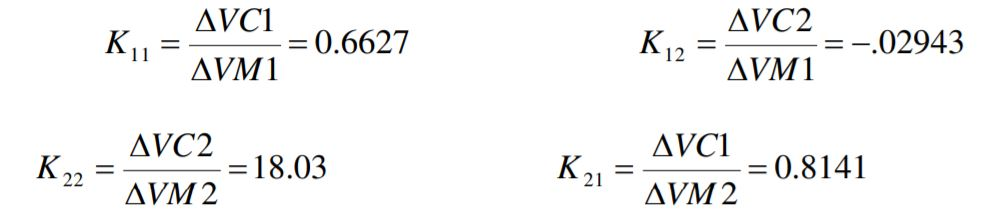Kij: gains of the process, where, i: is the controlled variable, j: is the

The relative gain is calculated from the steady-state gains of the process and cross-loop interaction models, which best describe the dynamic behavior of the process.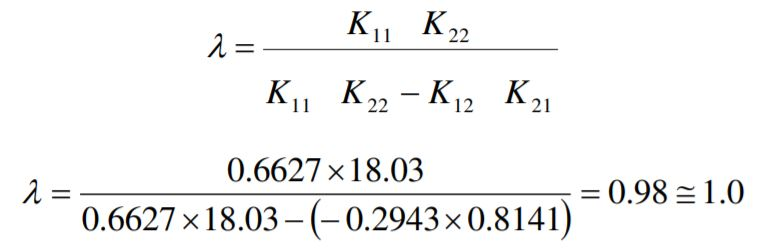When the relative gain is between 0.5 and 1, the interaction of the loops causes every action of the controllers to be reflected and amplified in both process variables. This is not surprising since the relative gain is positive and close to one. One method of control choice would be to use decouplers for each control loop, or to tune each controller separately by doing a simple decoupling .

Tuning using the BTL (biggest log-modulus) method.

One of the biggest questions in multivariable control is how to tune the controllers together with the interaction loop. If you have a PI controller, you have 2N (Kc, τI) parameters to select, if you have a PID, you have 3N (K cI, τD) parameters to select. BLT (Biggest Log-Modulus) has a standard tuning methodology. It meets the objective of arriving at a reasonable and optimal controller configuration with only a small amount of computation and computational help.

The method is based on the classical Ziegler-Nichols SISO method. Using the Nichols plot the controller is tuned by passing the Nichols curve tangentially through its respective dB curve, for a SISO system it corresponds to the +2 dB curve, for a 2x2 MIMO system the +4 dB curve and for a 3x3 MIMO system by the +6 dB curve . As the control strategy is a simple decoupling, the corresponding curve for its tuning is the +2 dB curve.

To obtain the initial parameters, the IMC tuning method was used for each loop, taking into account only the direct process. Table 2 presents a summary of the proposed calculations.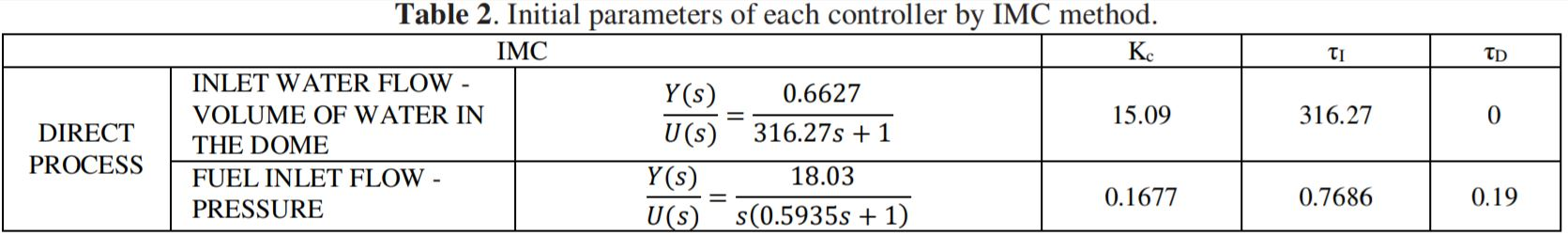A +2db tuning for Level control.

The main criterion of the BLT tuning method is to find the controller parameters that when plotting the open- loop transfer function tangentially pass through the +2dB curve. To find the controller parameters a factor was determined for each parameter (Kc, τI y τD)and with this to see its behavior in Nichols diagram. Figure 4 and Table 3 show the influence of the proportional gain (Kc) in the Nichols diagram for the level controller (τI and τD values remain constant).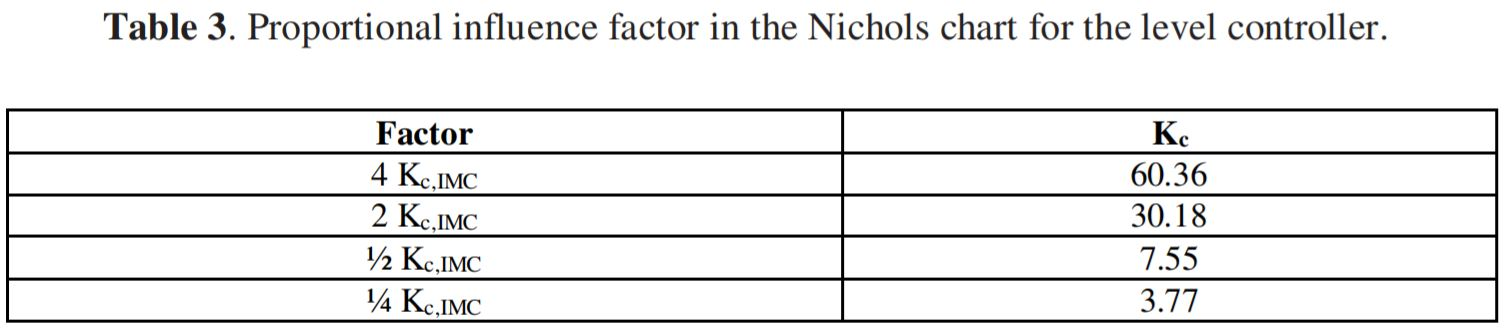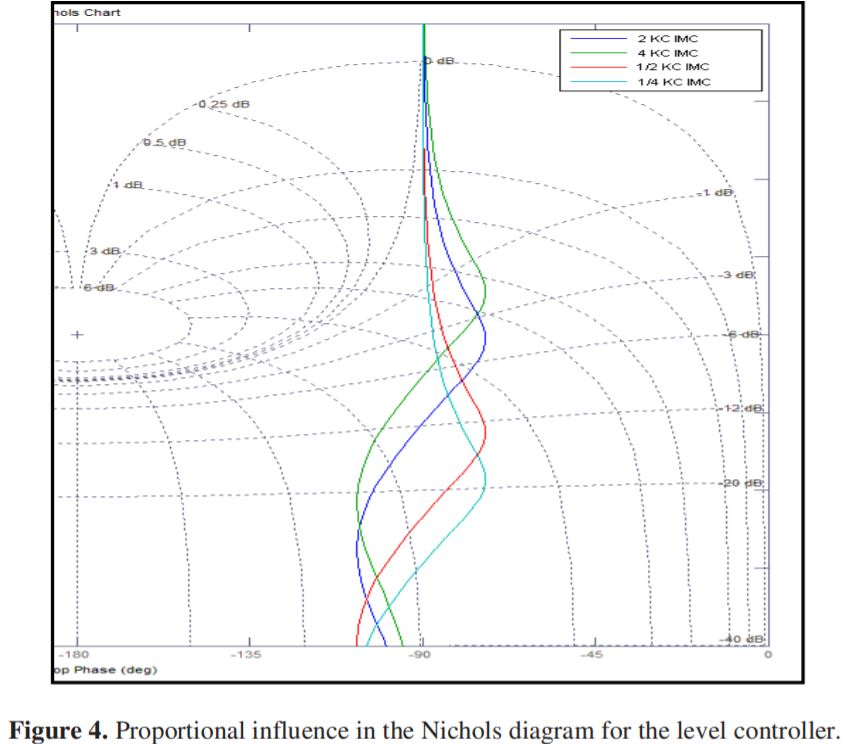Likewise, the influence of the integral time (τI) in the Nichols chart for the level controller was analyzed, the values of Kc =15.09 and τD =0 (table 4 and figure 5). On the other hand, Table 5 and Figure 6 show the influence of the derivative time (τD) on the Nichols chart for the level controller.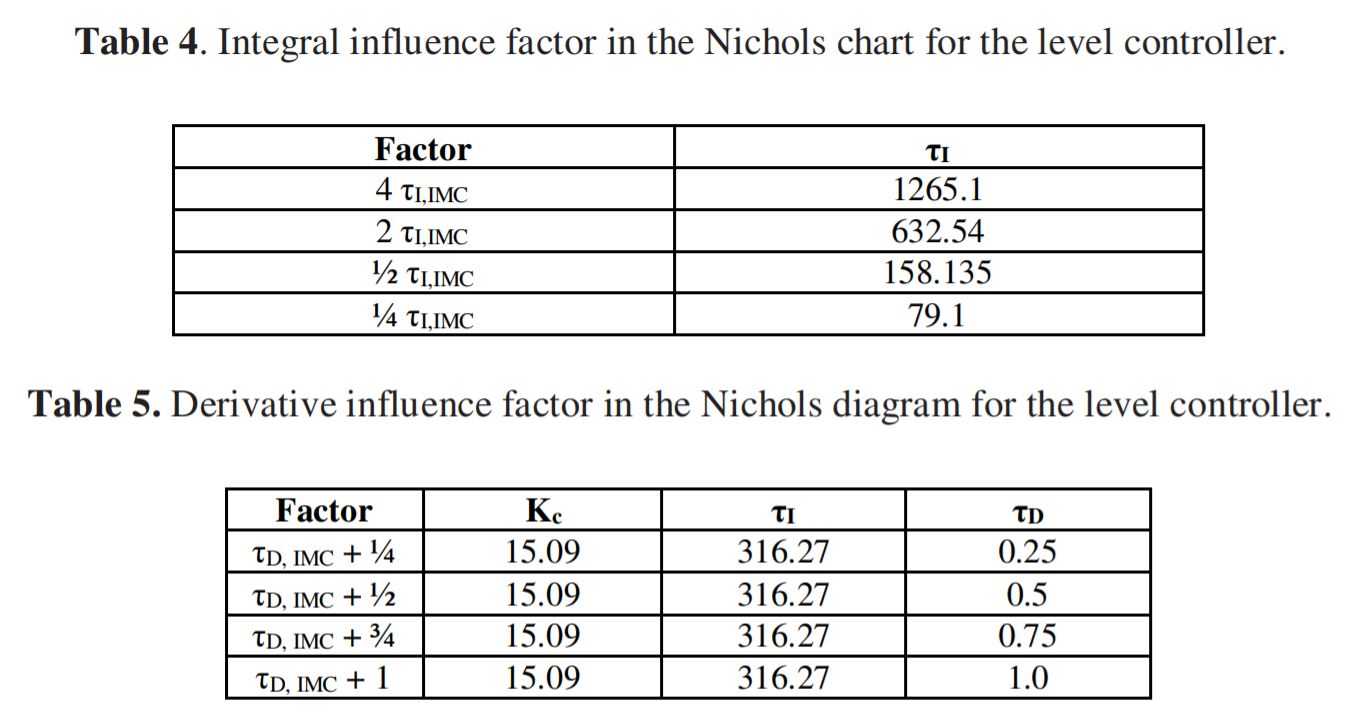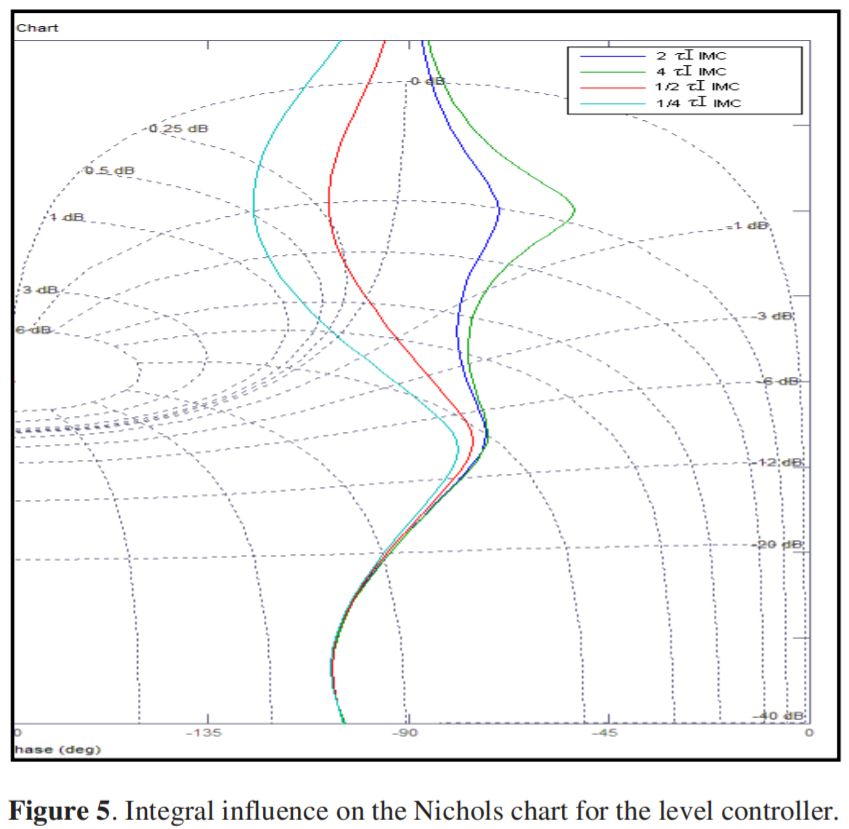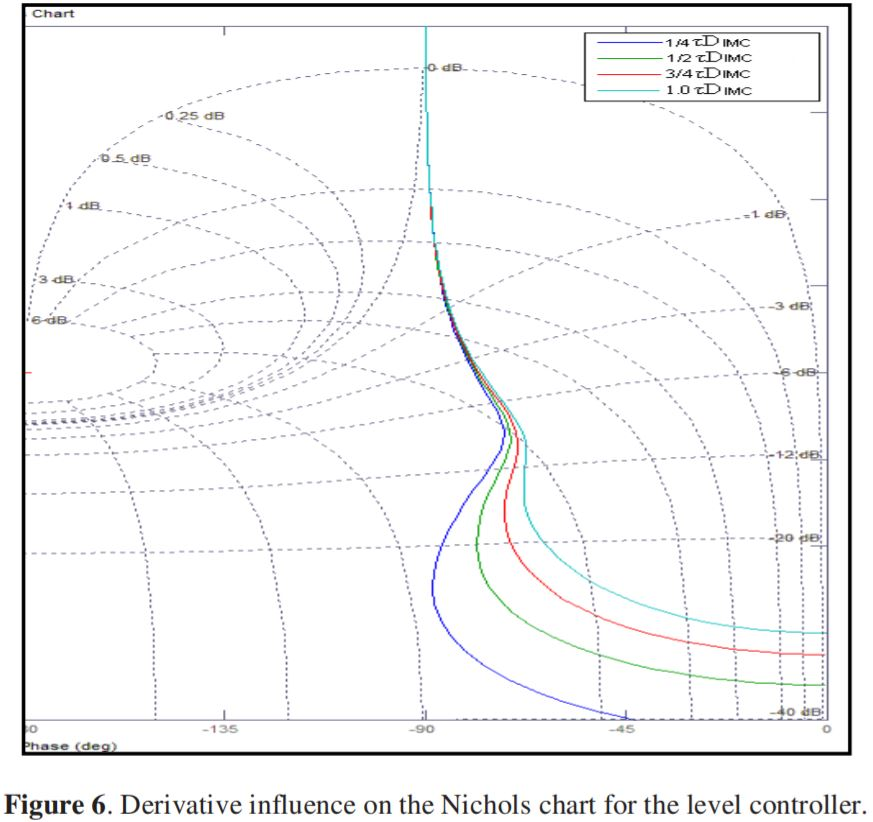BLT tuning using the Nichols chart at +2dB for the level controller.

From Figures 4, 5 and 6 it was determined which parameters to modify the PID controller for the level loop to tangentially pass through the +2 dB curve (Figure 7). Finding two options (Table 6).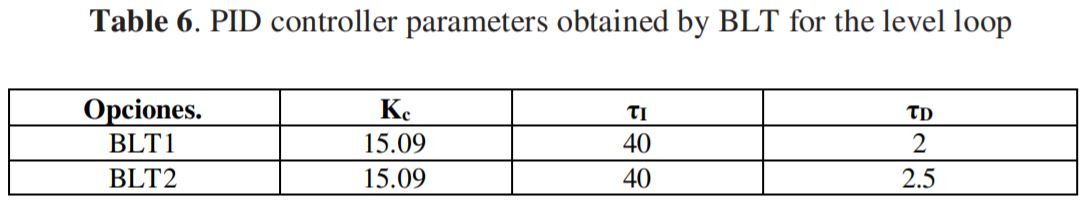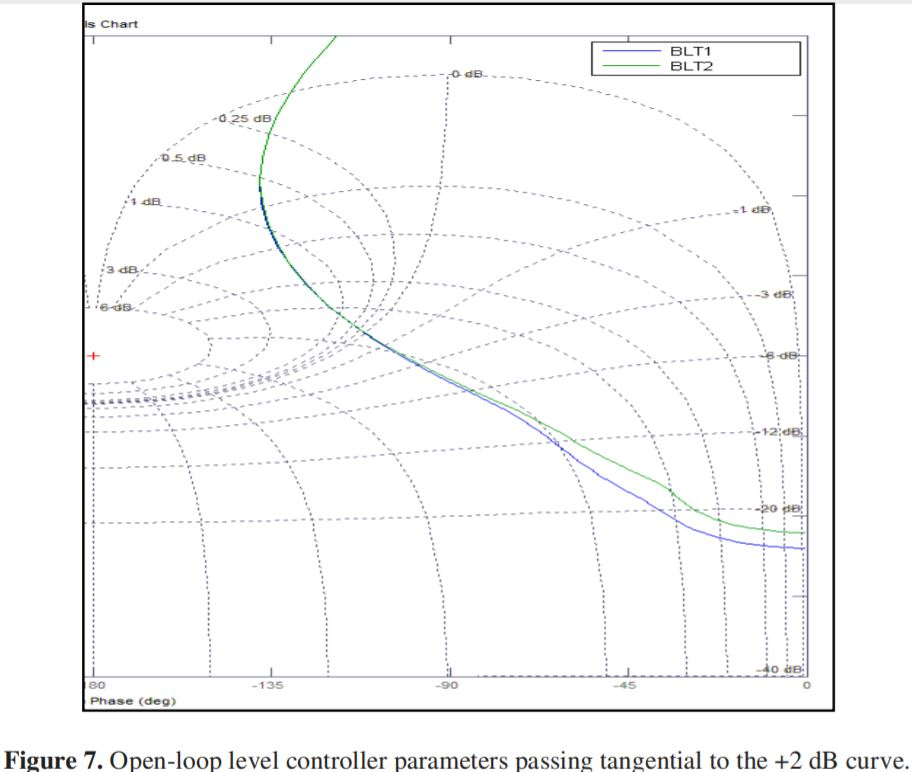Nichols adjustment to +2db for the pressure loop.

As in the level loop, factors are determined to modify the pressure controller parameters. Tables 7 to 10 show the results for PID control.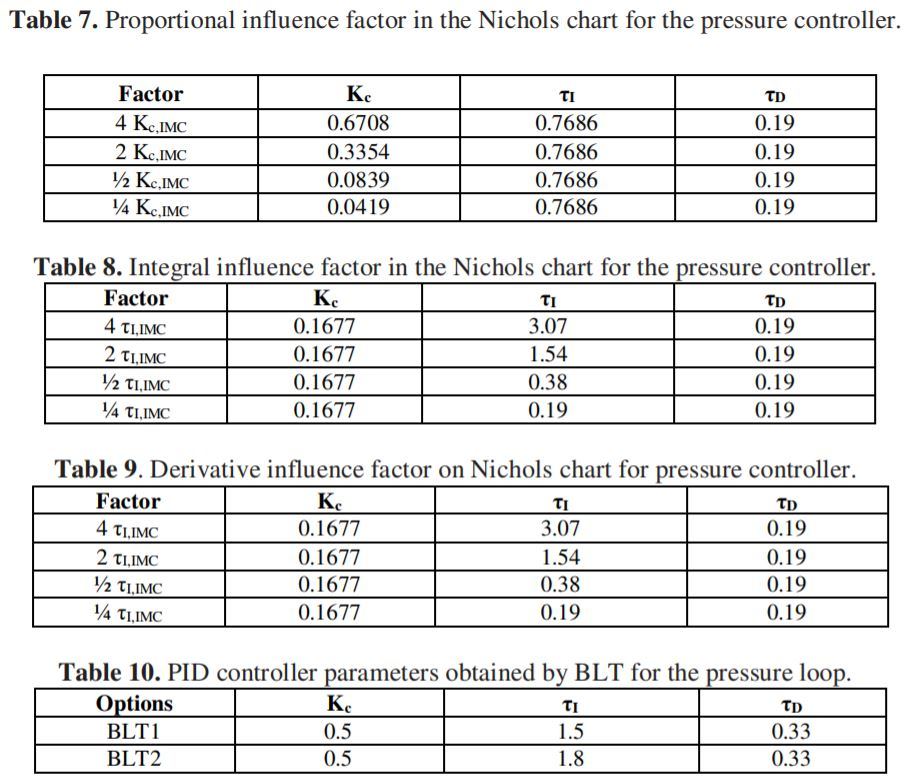Final parameters obtained by the blt method for the level (B11) and pressure (B22). controllers.

Table 11 shows the parameters obtained by the BLT method for each controller, which tangentially pass through the +2 dB curve in the Nichols diagram.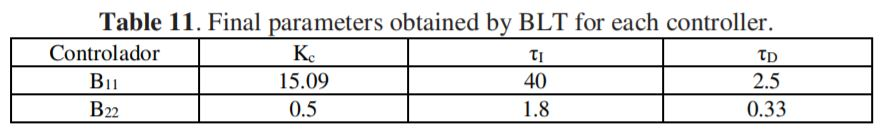Simulation in the Control Station Software.

With the parameters of the two controllers obtained by the BLT method, it is tested in the Control Station Software in 2X2 simulation, to observe the response time of each of the variables with their respective controller. By means of a step change. And thus retune to obtain optimal performance of each of the two controllers and minimize its reflection in each process. The two controllers are activated in automatic with the parameters obtained by the BLT method and each parameter is modified as follows.

Level controller.

Influence of the proportional gain on the response of the PID controller to a step change of 27.8% in the setpoint.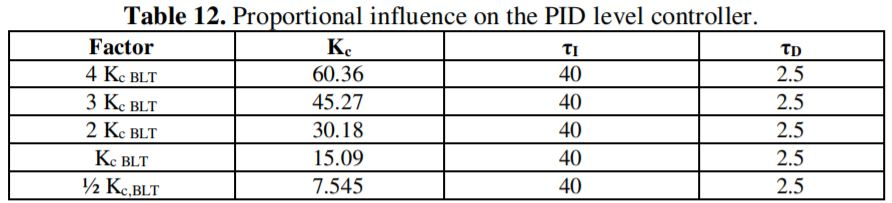As can be seen in Figure 8 and Table 13, as Kc increases the overshoot and oscillation decreases until it disappears, as is the case for 4 Kc BLT.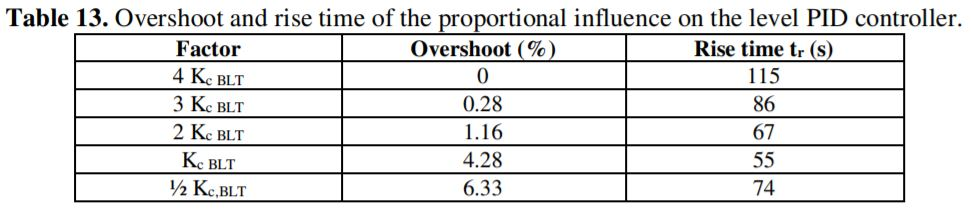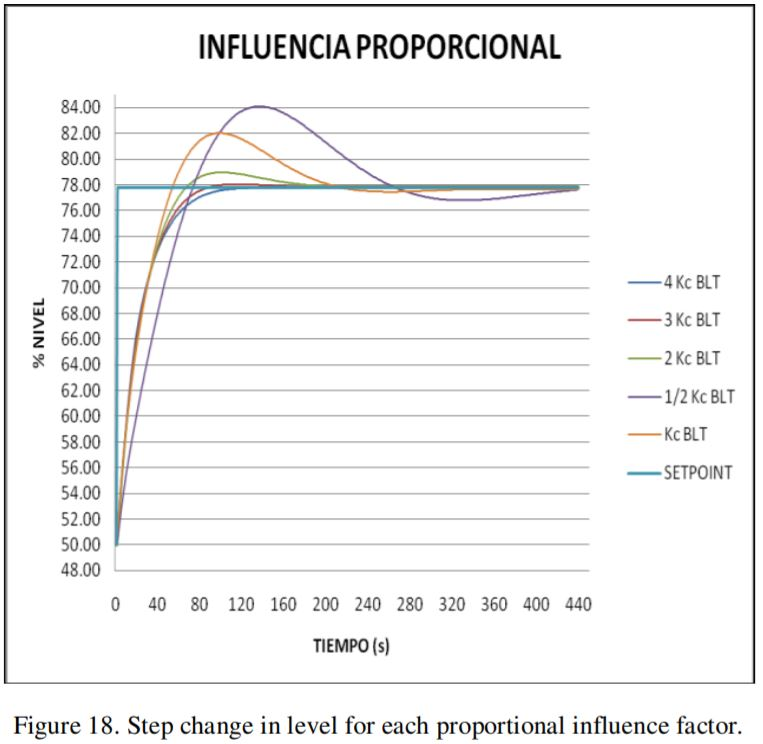Influence of the integral time on the PID controller response to a 27.8% step change in setpoint.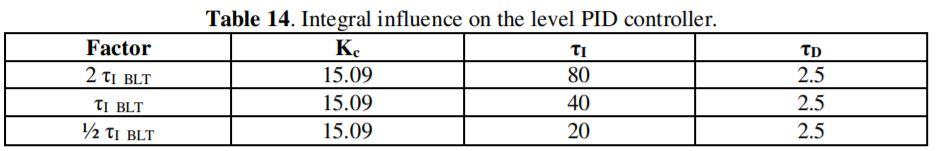As can be seen in Figure 9 and Table 15, as τI, decreases, the rise time decreases but the overshoot increases. On the contrary, when τI, is increased, the rise time increases, making the response slower but with less overshoot.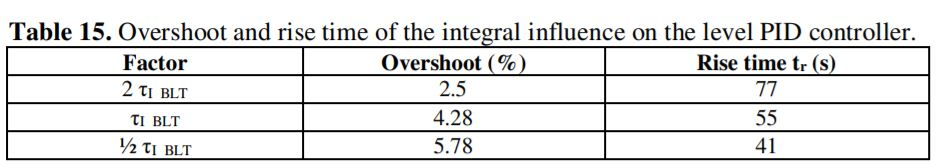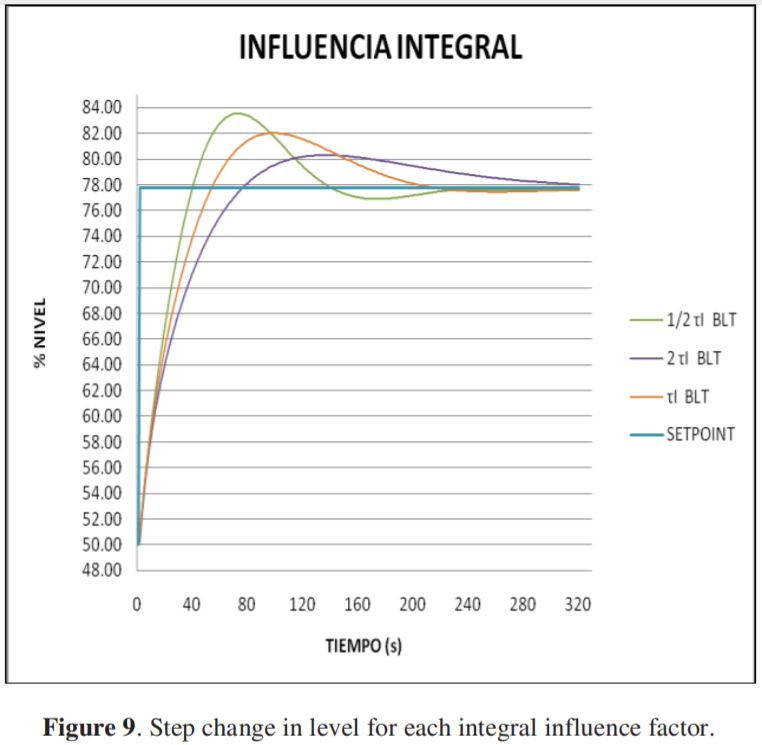Influence of the derivative time on the PID controller response to a 27.8% step change in setpoint.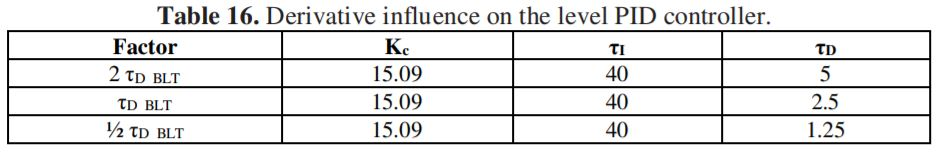As can be seen in Figure 10 and Table 17, as τD decreases, the rise time increases but the overshoot decreases. On the other hand, when τD is increased, the rise time decreases but the overshoot increases.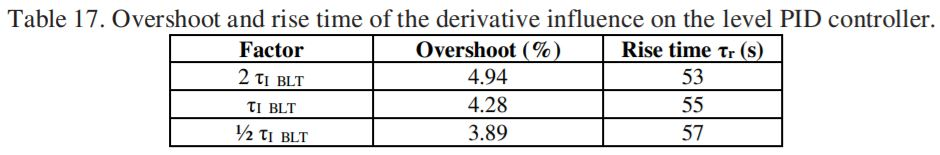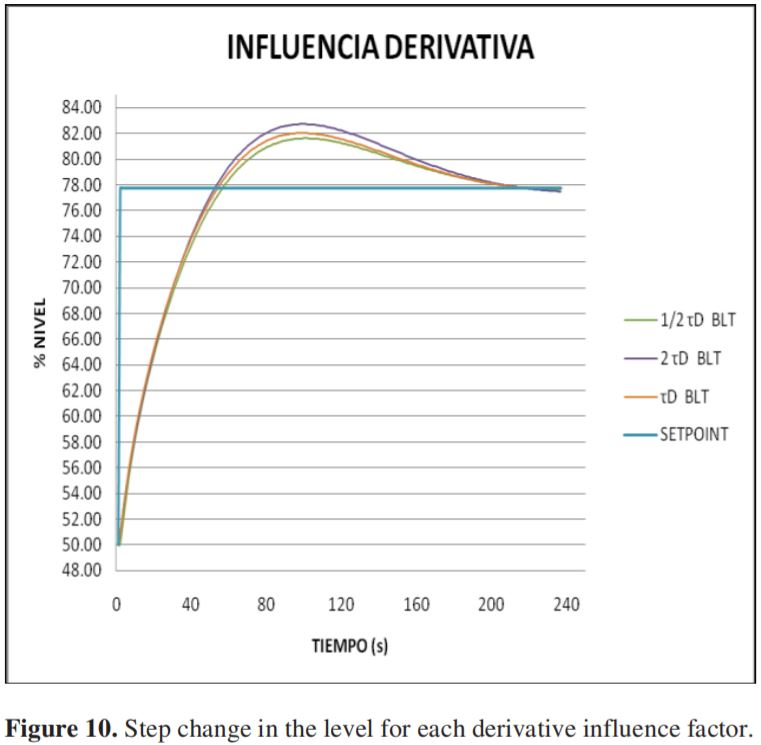For its tuning, the criterion of decreasing τI was taken to reduce the rise time as much as possible, but as can be seen, the shorter the integral time, the greater the overshoot, to counteract this, the proportional gain (Kc) was increased up to 4 times and the derivative time was decreased to ¼. With these new parameters (Table 19), a double rectangular pulse type test is performed, at the setpoint, with a magnitude of 27.8% and a total duration of 400 seconds, to observe the behavior of its main process variable (%Level), its interaction in the second pressure control loop and its reflection in each of the variables.

Analysis and choice of the parameters of the level controller.

As can be seen in Figure 11, B11 does not present overshoot and its time to reach its first setpoint (27.8% more) is 156 seconds, after the second change, it reaches its second setpoint (55.56% less) at 191 seconds. B12 has a very low overshoot (0.11%) that is corrected at 172 seconds, its time to the first setpoint is 95 seconds and to the second is 197 seconds. B13 has a high overshoot (0.44%) that is corrected at 140 seconds, reaches the first setpoint at 59 seconds after the first change and reaches its second setpoint at 175 seconds after the change.

The final parameters of controller 1 (B11) are those of B12 where it has a very low overshoot (0.11%) and its response time is fast. Its reflection in the pressure control variable is not significant (0.75 Kpa). And its changes in the manipulated variable are smooth and gradual compared to the other 2 options.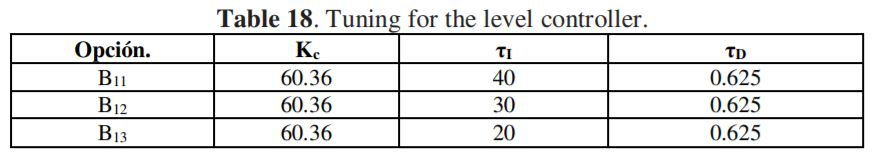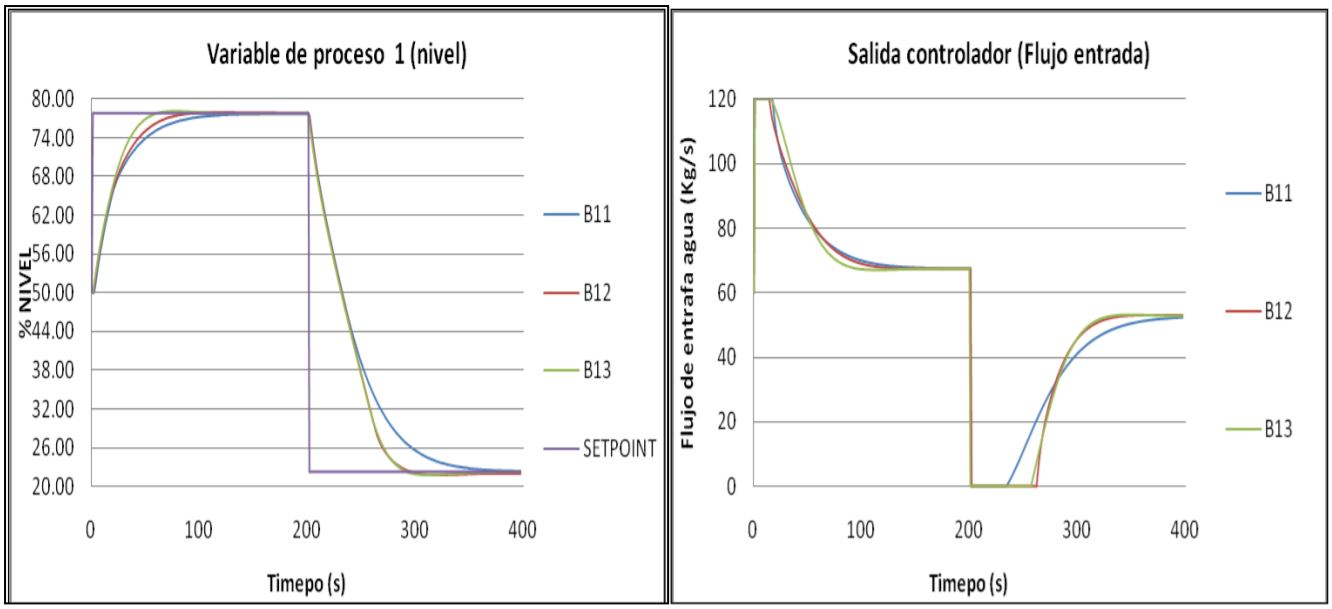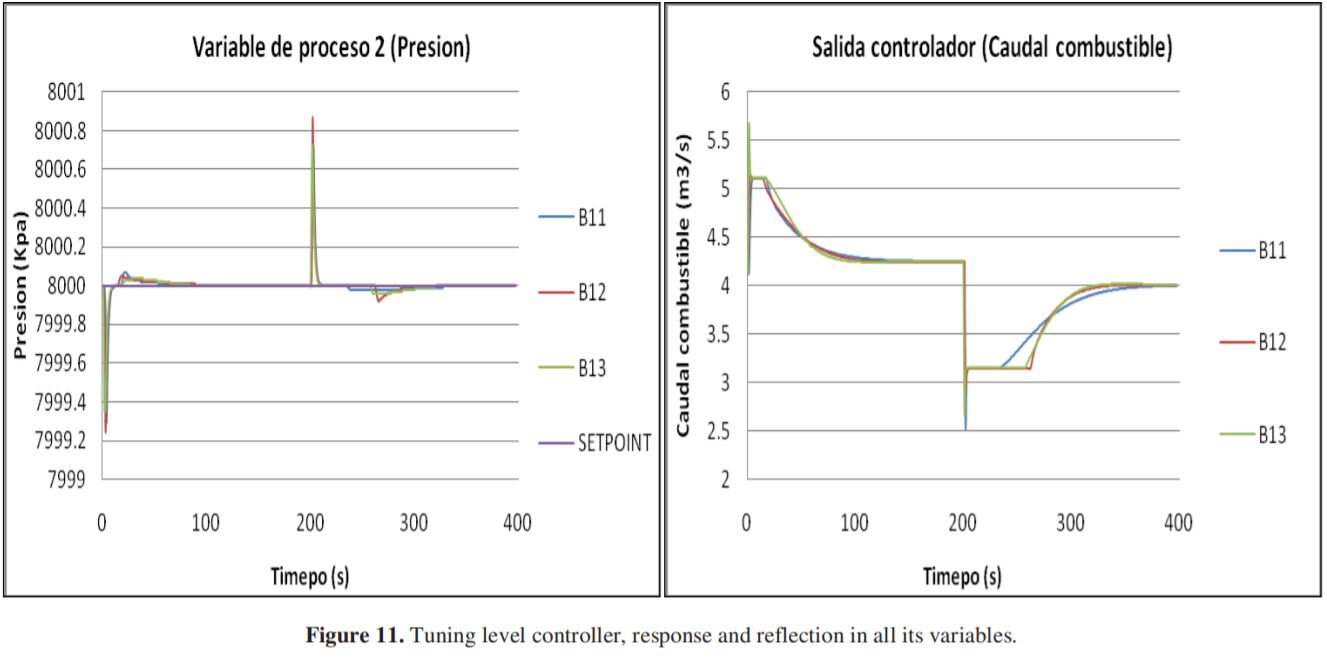Pressure controller.

The results of the influence of the proportional gain on the response of the PID controller to a step change of 1000 Kpa in the setpoint are presented (Table 19).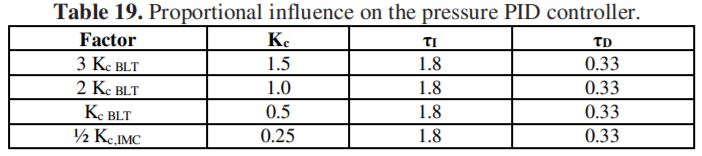As can be seen in Figure 12 and Table 20, as Kc increases the rise time decreases, although the decrease is not very significant. As the gain increases up to 3KcBLT there is an oscillation of 0.11 Kpa above setpoint and 0.07 below which is not corrected.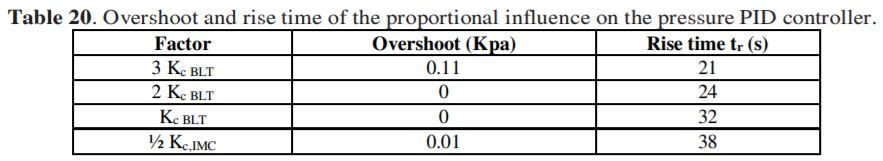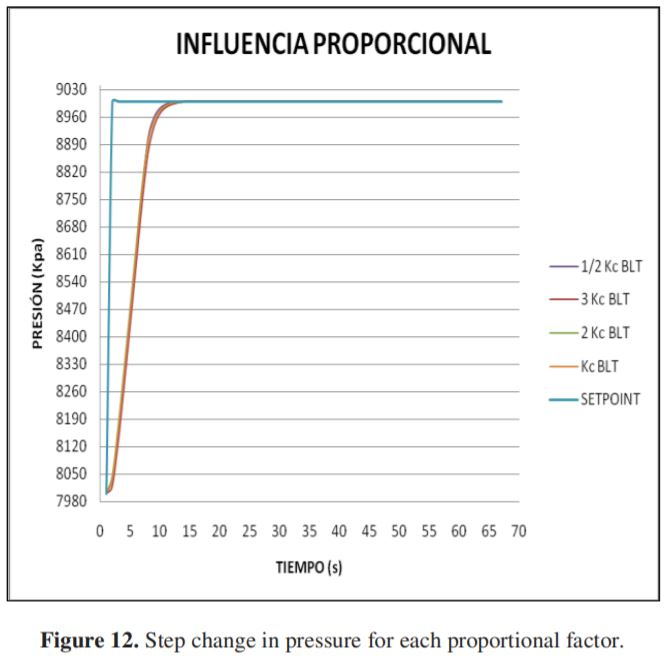Influence of integral time on the PID controller response to a step change of 1000 Kpa at the setpoint (Table 21).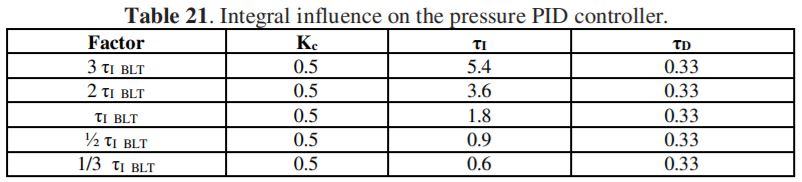As can be seen in Figure 13 and Table 22, as τI decreases the rise time decreases significantly, but increases the overshoot more and more.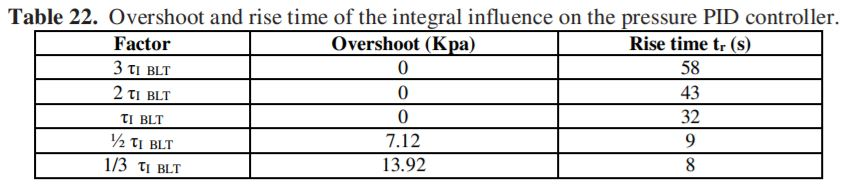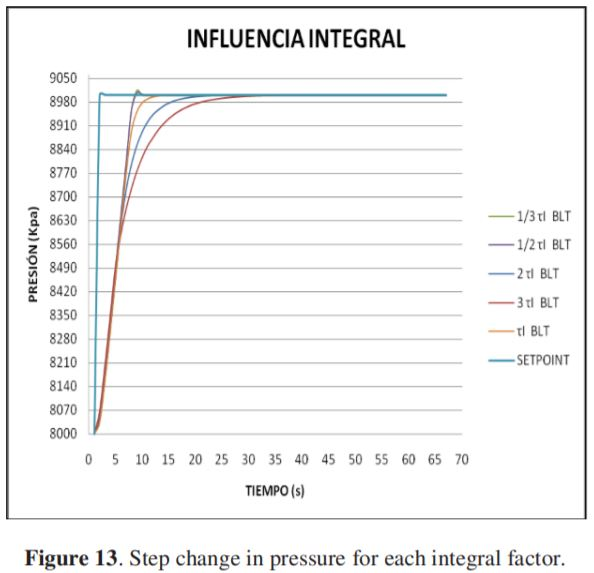Influence of the derivative time on the response of the PID controller to a step change of 1000 Kpa at the setpoint. (Table 23).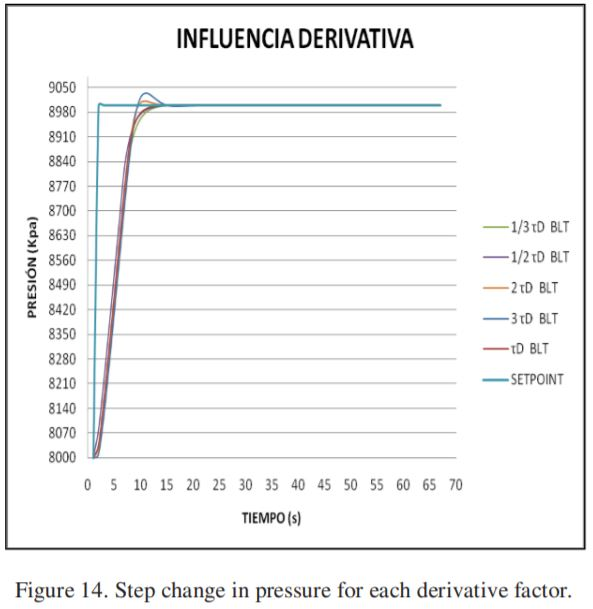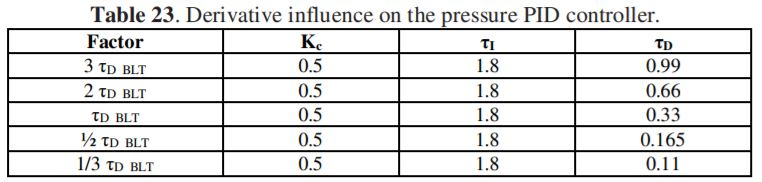As can be seen in Figure 14 and Table 24, as τD decreases, the rise time increases and there is no overshoot. On the contrary, when it is increased, a significant decrease in the rise time is seen but an overshoot is generated that is increasingly larger.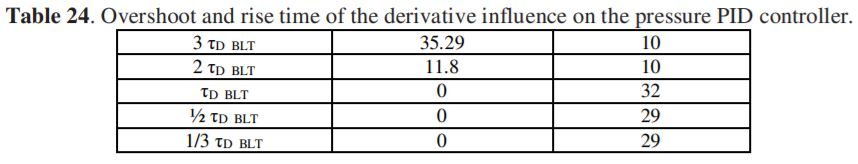For its tuning, the criterion of decreasing τI was taken to reduce the rise time to the maximum, but as it can be seen that the shorter the integral time the greater the overshoot, to counteract this, Kc is increased up to 2 times and the derivative time obtained by BLT is left (Table 25).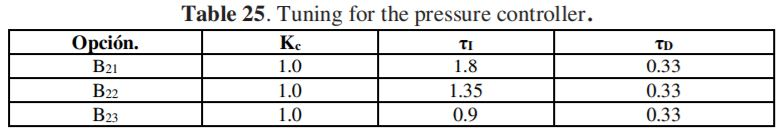A double rectangular pulse type test was performed at the setpoint, with a magnitude of 1000 Kpa and 357 seconds of duration, to observe the behavior of its main process variable (pressure) and its interaction in the level loop, and its reflection in each of the variables.

Analysis and choice of the parameters of the pressure controller, as can be seen in Figure 15, the three graphs are very similar and differ in a few seconds to obtain the desired value (setpoint), B21 reaches the setpoint at 65 of the first change (1000 Kpa more), at 91 seconds reaches its second setpoint (2000 Kpa less) after this, B22 and B23 obtain the first setpoint at 61 and 54 seconds respectively, and the second at 285 and 278.

The final parameters of controller 2 are those of B23 since its response speed is the most optimal and does not present overshoot. Table 26 shows the final parameters of each controller obtained using the criteria mentioned above.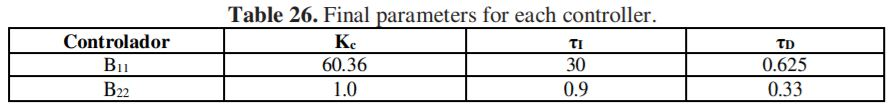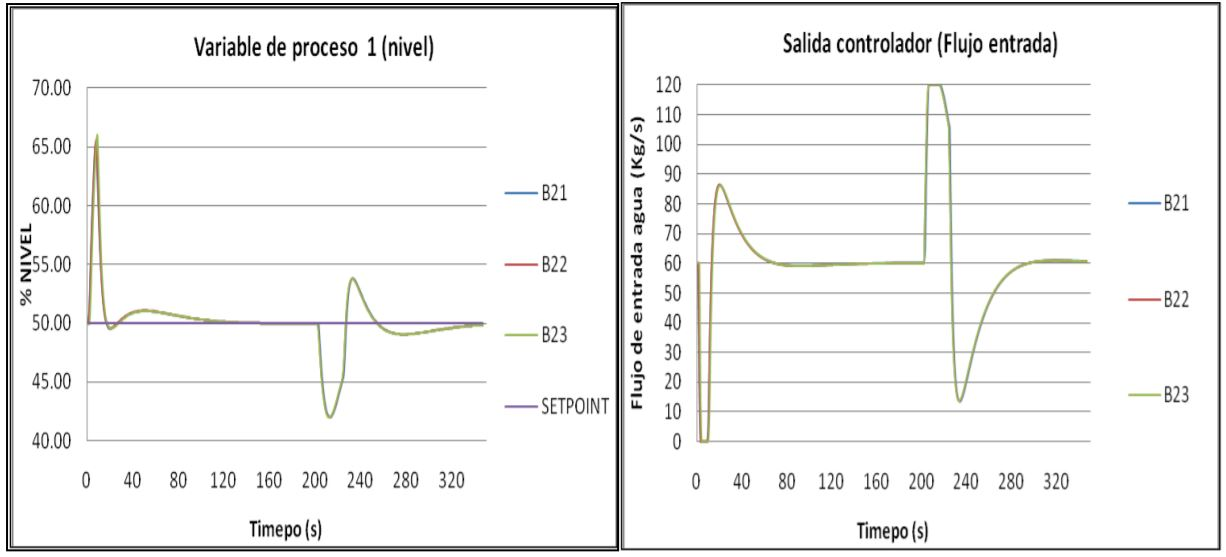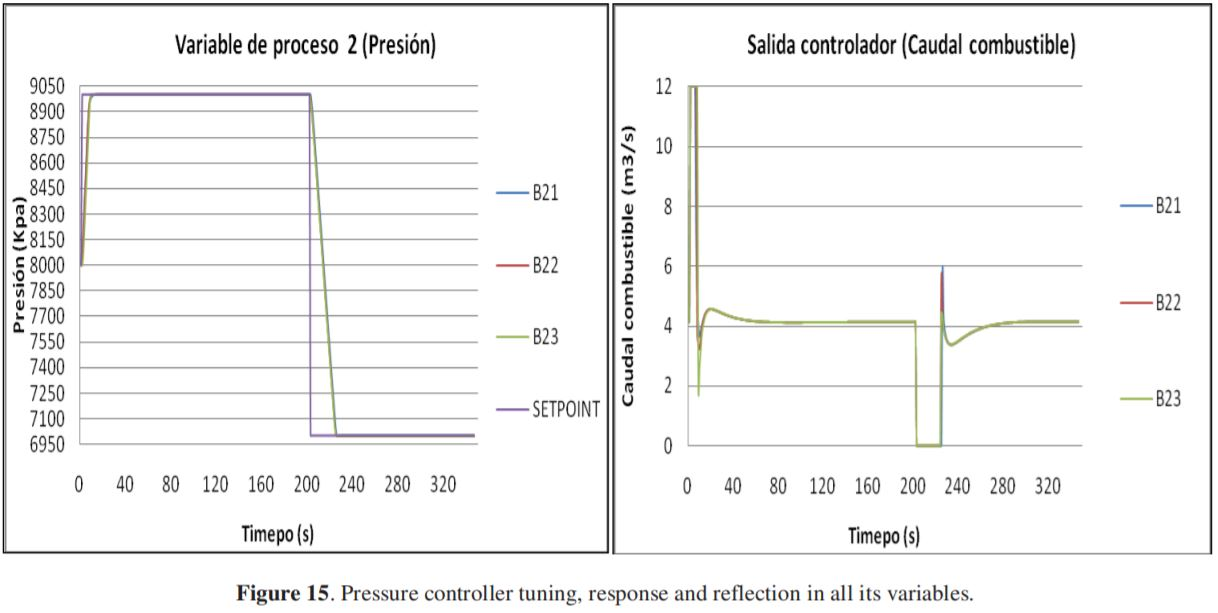DISTURBANCE IN THE REGULATING SYSTEM. VARIABLE DISTURBANCE STEAM OUTPUT FLOW (Kg/s).

The BLT tuning and the tuning performed in Control Station were performed for changes in the setpoint of each controller (controller 1 - level and controller 2 - pressure) keeping the disturbance variable constant. But changes due to increase or decrease in steam demand is also a variable that affects the level in the dome and with greater influence the outlet pressure.

Since a control system is rarely tuned for change in the setpoint and in turn for disturbance, the setpoint was left stable and the system was applied to a double rectangular pulse disturbance of magnitude 40 Kg / s to see the response of the controllers with the final parameters and all system variables.

As can be seen in Figure 16, for a magnitude as high as 40 Kg/s, the controllers responded quickly, not allowing the level to increase by more than 1.06% and decrease by 2.22%. For the pressure, there was a decrease with respect to the setpoint of 0.01% and an increase of 0.02%. These changes were corrected in a matter of seconds, obtaining all variables in the desired state.

Conclusions

The final parameters of each controller are used for servo control and regulatory control in the 2x2 MIMO system, thus allowing a wide application in the operating conditions and minimizing the effects of each disturbance and its reflection in each variable.

The transfer functions obtained by the Control Station software, by means of double rectangular pulse type perturbations, have a high degree of approximation to the dynamics that normally occurs in the dome of a water-tube boiler obtained from the boiler software.

The stability criteria applied (Nichols and Nyquist) allow verifying that the control loops simulated in automatic are stable.

The pressure-controlled variable (P) responds faster and reaches its new setpoint in less time than the level controlled variable (NW).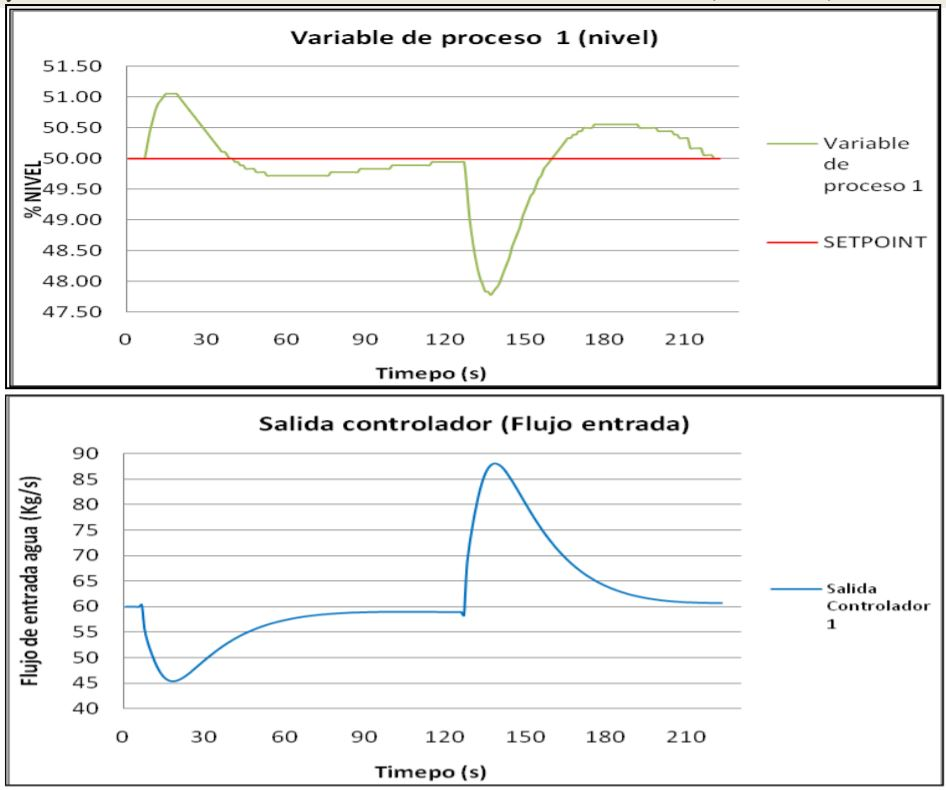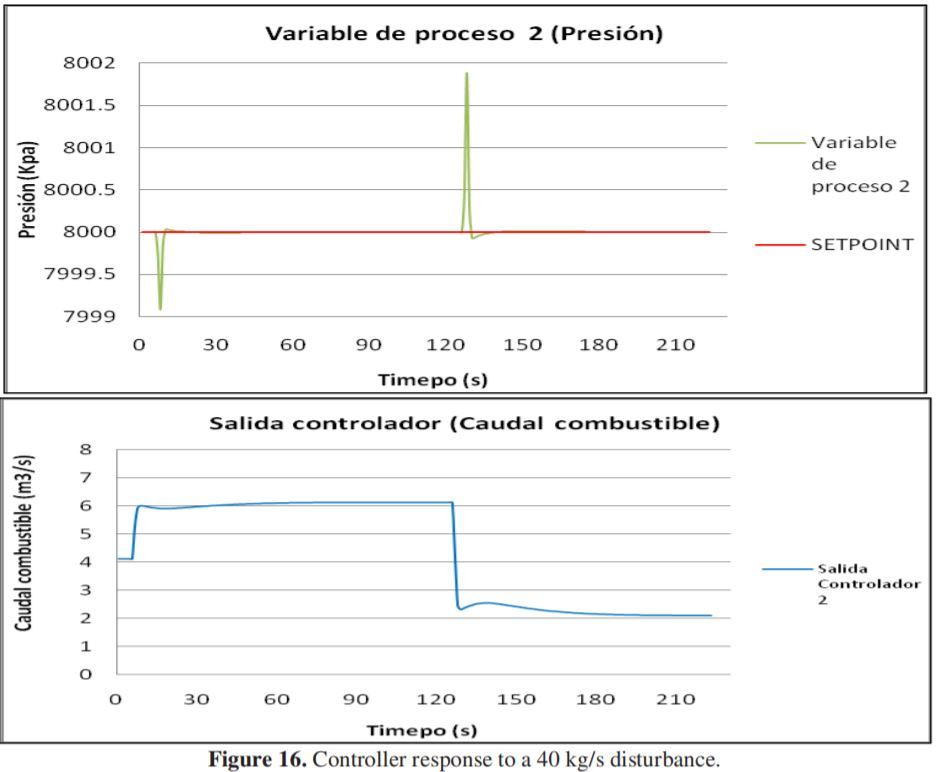References

 F. Bonilla Macuil, J. C., Zavala González, “Construcción de un generador de vapor con capacidad para 7.5 kg/hr de gasto a 10 Bar. de presión y 220oc y manual de uso, reglas de seguridad y mantenimiento del mismo; así como prácticas de laboratorio,” Universidad de las Américas, Puebla, 2003.

 I. M. Chew, F. Wong, A. Bono, J. Nandong, and K. I. Wong, “Performance Analysis of the Level Control with Inverse Response by using Particle Swarm Optimization,” in Lecture Notes in Electrical Engineering, vol. 603, pp. 55–64, 2020. doi: 10.1007/978-981-15-0058-9_6.

 D. Cooper, J. Arbogast, and R. Jyringi, “Modeling, analysis and tuning tools for teaching process dynamics and control,” in AIChE Annual Meeting, Conference Proceedings, pp. 3566, 2005.

 C. D. Rocco and R. Morabito, “Um modelo de otimização para as operações de produção de vapor em caldeiras industrials,” Gestao e Producao, 2012, doi: 10.1590/S0104-530X2012000200004.

 A. Serbezov and D. Cummings, “University-Industry Co-Operation to Promote Industrial Relevance in the Field Instrumentation Component of Control Education,” IFAC-PapersOnLine, vol. 49, no. 6, pp. 309–313, 2016, doi: 10.1016/j.ifacol.2016.07.195.

 A. Serbezov and R. Rice, “Imparting Industrially Relevant Process Control Skills in Chemical Engineering Students with LOOP-PRO TRAINER,” in IFAC-PapersOnLine, vol. 52, no. 9, pp. 33–35, 2019. doi: 10.1016/j.ifacol.2019.08.118.

 H. He and Y. Tan, “An optimizing parameter-tuning of multi-loop controllers for boiler combustion process,” in Proceedings of the 2nd IASTED Asian Conference on Modelling, Identification, and Control, AsiaMIC 2012, pp. 78–83, 2012. doi: 10.2316/P.2012.769-029.

 J. Gaines and D. Nash, “Batch control gets easier,” Chemical Processing, vol. 72, no. 2, pp. 39–41, Feb. 2009.

 H. Pan, W. Zhong, Z. Wang, and G. Wang, “Optimization of industrial boiler combustion control system based on genetic algorithm,” Computers and Electrical Engineering, vol. 70, pp. 987–997, Aug. 2018,. doi: 10.1016/j.compeleceng.2018.03.003.

 V. Köppel, M. Segal, and M. Remer, “Análisis de Estrategias de Desacoplamiento en Sistemas Interactuantes de Control de Procesos,” Informacion Tecnologica, 2004,. doi: 10.4067/s0718- 07642004000600013.

 J. Moreno Lemos and R. A. Trujillo Perez, “Simulación dinámica y estrategias de control en el domo de una caldera acuatubular,” Universidad del Valle, 2006.

 I. M. Chew, F. Wong, A. Bono, J. Nandong, and K. I. Wong, “Feedforward plus feedback control scheme and computational optimization analysis for integrating process,” in Lecture Notes in Electrical Engineering, vol. 481, pp. 359–368, 2019. doi: 10.1007/978-981-13-2622-6_35.

 F. G. Shinskey, “Process Control: As Taught vs as Practiced,” Industrial & Engineering Chemistry Research, vol. 41, no. 16, pp. 3745–3750, Aug. 2002,. doi: 10.1021/ie010645n.

 S. C. A. & C. B.Armando, “Principles and practice of automatic process control,” Automatica, 1997.

[15 T. E. Marlin, “The software laboratory for undergraduate process control education,” Computers & Chemical Engineering, vol. 20, no. SUPPL.2, pp. S1371–S1376, Jan. 1996,. doi: 10.1016/0098- 1354(96)00235-9.

 D. J. Cooper, “Practical Process Control using Loop-Pro Software,” Control Station Inc., 2005.

 W. L. LUYBEN, “Process modeling, simulation and control for chemical engineers, 2 ed”,. Mc Graw Hill, 1990.